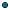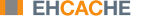About Ehcache : Why Caching Works : How Much Will an Application Speed up with Caching?
How Much Will an Application Speed up with Caching?
In applications that are I/O bound, which is most business applications, most of the response time is getting data from a database. In a system where each piece of data is used only one time, there is no benefit. In a system where a high proportion of the data is reused, the speed up is significant.
Applying Amdahl's Law
Amdahl’s law finds the total system speedup from a speedup in part of the system.
1 / ((1 - Proportion Sped Up) + Proportion Sped Up / Speed up)
The following examples show how to apply Amdahl’s law to common situations. In the interests of simplicity, we assume:A single server.A system with a single thing in it, which when cached, gets 100% cache hits and lives forever.
Persistent Object Relational Caching
A Hibernate Session.load() for a single object is about 1000 times faster from cache than from a database. .
A typical Hibernate query will return a list of IDs from the database, and then attempt to load each. If Session.iterate() is used, Hibernate goes back to the database to load each object.
Imagine a scenario where we execute a query against the database that returns a hundred IDs and then loads each one. The query takes 20% of the time and the round trip loading takes the rest (80%). The database query itself is 75% of the time that the operation takes. The proportion being sped up is thus 60% (75% * 80%).
The expected system speedup is thus:
1 / ((1 - .6) + .6 / 1000)
= 1 / (.4 + .0006)
= 2.5 times system speedup
Web Page Caching
An observed speed up from caching a web page is 1000 times. Ehcache can retrieve a page from its SimplePageCachingFilter in a few milliseconds.
Because the web page is the result of a computation, it has a proportion of 100%.
The expected system speedup is thus:
1 / ((1 - 1) + 1 / 1000)
= 1 / (0 + .0001)
= 1000 times system speedup
Web Page Fragment Caching
Caching the entire page is a big win. Sometimes the liveness requirements vary in different parts of the page. Here the SimplePageFragmentCachingFilter can be used.
Let's say we have a 1000 fold improvement on a page fragment that taking 40% of the page render time.
The expected system speedup is thus:
1 / ((1 - .4) + .4 / 1000)
= 1 / (.6 + .0004)
= 1.6 times system speedup
Cache Efficiency
Cache entries do not live forever. Some examples that come close are:Static web pages or web page fragments, like page footers.Database reference data, such as the currencies in the world.
Factors that affect the efficiency of a cache are:Liveliness — How live the data needs to be. The less live, the more it can be cachedProportion of data cached — What proportion of the data can fit into the resource limits of the machine. For 32-bit Java systems, there was a hard limit of 2 GB of address space. 64-bit systems do not have that constraint, but garbage collection issues often make it impractical to have the Java heap be large. Various eviction algorithms are used to evict excess entries.Shape of the usage distribution — If only 300 out of 3000 entries can be cached, but the Pareto (80/20 rule) distribution applies, it might be that 80% of the time, those 300 will be the ones requested. This drives up the average request lifespan.Read/Write ratio — The proportion of times data is read compared with how often it is written. Things such as the number of empty rooms in a hotel change often, and will be written to frequently. However the details of a room, such as number of beds, are immutable, and therefore a maximum write of 1 might have thousands of reads.
Ehcache keeps these statistics for each cache and each element, so they can be measured directly rather than estimated.
Cluster Efficiency
Assume a round-robin load balancer where each hit goes to the next server. The cache has one entry which has a variable lifespan of requests, say caused by a time to live (TTL) setting. The following table shows how that lifespan can affect hits and misses.
Server 1 Server 2 Server 3 Server 4
M M M M
H H H H
H H H H
H H H H
H H H H
... ... ... ...
The cache hit ratios for the system as a whole are as follows:
Entry
Lifespan Hit Ratio Hit Ratio Hit Ratio Hit Ratio
in Hits 1 Server 2 Servers 3 Servers 4 Servers
2 1/2 0/2 0/2 0/2
4 3/4 2/4 1/4 0/4
10 9/10 8/10 7/10 6/10
20 19/20 18/20 17/20 16/10
50 49/50 48/50 47/20 46/50
The efficiency of a cluster of standalone caches is generally:
(Lifespan in requests - Number of Standalone Caches) / Lifespan in requests
Where the lifespan is large relative to the number of standalone caches, cache efficiency is not much affected. However when the lifespan is short, cache efficiency is dramatically affected. This problem can be solved using the distributed caching capability provided in Terracotta BigMemory Max. With distributed cache, entries put into a local cache are propagated to other servers in the cluster.
A Cache Version of Amdahl's Law
Applying Amdahl's law to caching, we now have:
1 / ((1 - Proportion Sped Up * effective cache efficiency) +
(Proportion Sped Up * effective cache efficiency)/ Speed up)
effective cache efficiency = (cache efficiency) * (cluster efficiency)
Web Page Example
Applying this formula to the earlier web page cache example where we have cache efficiency of 35% and average request lifespan of 10 requests and two servers:
cache efficiency = .35
cluster efficiency = .(10 - 1) / 10
= .9
effective cache efficiency = .35 * .9
= .315
1 / ((1 - 1 * .315) + 1 * .315 / 1000)
= 1 / (.685 + .000315)
= 1.45 times system speedup
If the cache efficiency is 70% (two servers):
cache efficiency = .70
cluster efficiency = .(10 - 1) / 10
= .9
effective cache efficiency = .70 * .9
= .63
1 / ((1 - 1 * .63) + 1 * .63 / 1000)
= 1 / (.37 + .00063)
= 2.69 times system speedup
If the cache efficiency is 90% (two servers):
cache efficiency = .90
cluster efficiency = .(10 - 1) / 10
= .9
effective cache efficiency = .9 * .9
= .81
1 / ((1 - 1 * .81) + 1 * .81 / 1000)
= 1 / (.19 + .00081)
= 5.24 times system speedup
The benefit is dramatic because Amdahl’s law is most sensitive to the proportion of the system that is sped up.|   Feedback